# Valuing Equity Interests in Complex Capital StructuresCase Studies

## In Part 2 of our series, we present case studies on applications of the four most widely selected valuation methods used for fair value measurement of multi-share capital structures.

November 14, 2019

See Part 1 of our series, Valuing Equity Interests in Complex Capital Structures: Common Valuation Approaches.

We present four case studies, herein, such that each demonstrates the practical use of one method discussed in Part 1 of this series. For demonstration, we provide a hypothetical, straightforward capital structure that will be used in all cases.

#### Figure 1. Capitalization Structure Assumptions### Probability-Weighted Expected Return Method

The PWERM method is most suitable when the company has realistic and reasonable assumptions on various future outcomes (e.g., IPO, M&A). Therefore, we take an IPO consideration with a one-year timeframe and a range of potential pre-money IPO exit equity values between \$50 million and \$100 million. This range is provided by the company and estimated based on discussions with the investment bankers and considering recent market transactions and respective industry multiples. First, we present a low equity value pre-IPO consideration below.

#### Figure 2. PWERM Analysis - IPO Scenario - Low Equity Value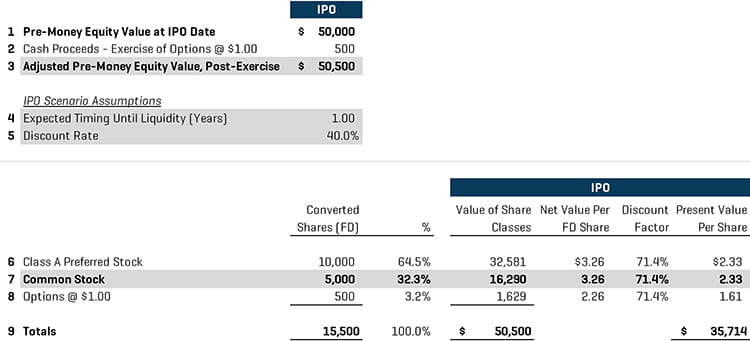In line 2 in Figure 2, we add proceeds from the exercise of any options being in the money to the pre-money IPO equity value and reach the adjusted pre-money and post-exercise equity value. For this case study, we assume a one-year expected term until IPO and an implied discount rate of 40%. Then, the equity value is allocated to the various tranches on a pro rata basis. Since this is an IPO scenario, the preferred stock is automatically converted to common stock equivalent shares. After applying the discount factor, we obtain the present value equivalent on a per share basis for each tranche. The same framework is applied to the high equity value consideration below, with the main difference being the starting equity value, which reflects the higher end of the pre-money IPO equity value range.

#### Figure 3. PWERM Analysis – IPO Scenario – High Equity Value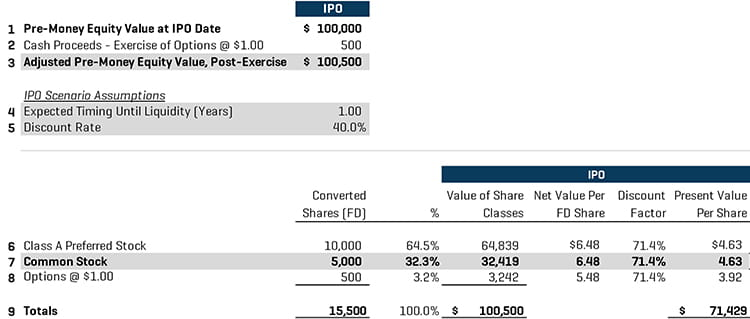After obtaining the present value equivalent on a per share basis for both low and high equity value considerations, we present the summary of the conclusions per security class and per scenario below.

#### Figure 4. Conclusion of Value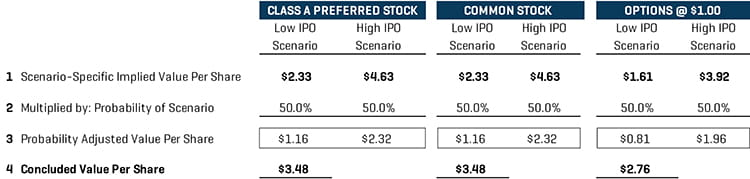After the application of the appropriate probability per scenario, the determined value per share is presented in line 4 of Figure 4.

### Option Pricing Method

The OPM is the most commonly used valuation method and recognizes the option-like payoff structure of the share classes. Following the same hypothetical capital structure, we present the first step of an OPM analysis below, which is the breakpoint analysis or strike prices outline, based on the fundamental structural privileges of the various equity units and their liquidation rights.

#### Figure 5. Breakpoint Analysis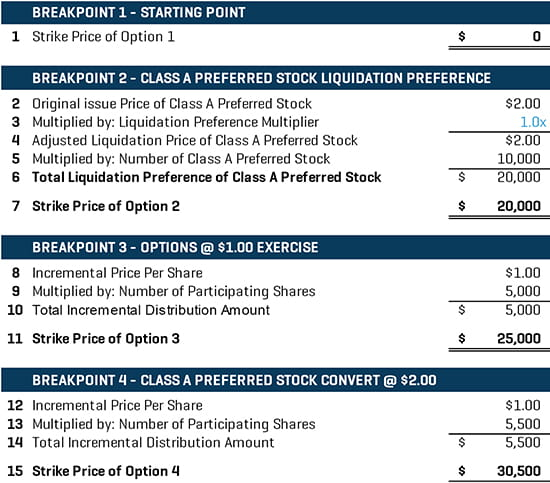The starting strike price is always \$0. The second breakpoint in this case reflects the liquidation preference of the Class A preferred stock, which has the first seniority in the distribution claims. The \$20 million in line 7 in Figure 5 reflects the total unpaid principal that needs to be repaid to the preferred stockholders. In this case, there is no dividend considered, and this breakpoint is just a reflection of the total unpaid capital commitment. If the equity value is higher than this threshold, then the common stock starts participating. The third breakpoint corresponds to the equity value threshold at which the holders Options @ \$1.00 start to exercise their options and participate in any further distribution of equity value. Between the second and third breakpoints, only the common stock holders participate on a pro rata basis up to a distribution amount of \$1.00 per share. The fourth breakpoint corresponds to the equity value threshold at which it is meaningful for the preferred stockholders to convert their shares into common shares and share the upside from a potential liquidity event. If the equity value at the end of the term is lower than this threshold, it is not beneficial for the holders to convert the shares into the common stock equivalent shares. After the breakpoint analysis, we present the allocation of equity value analysis below.

#### Figure 6. Allocation of Equity Value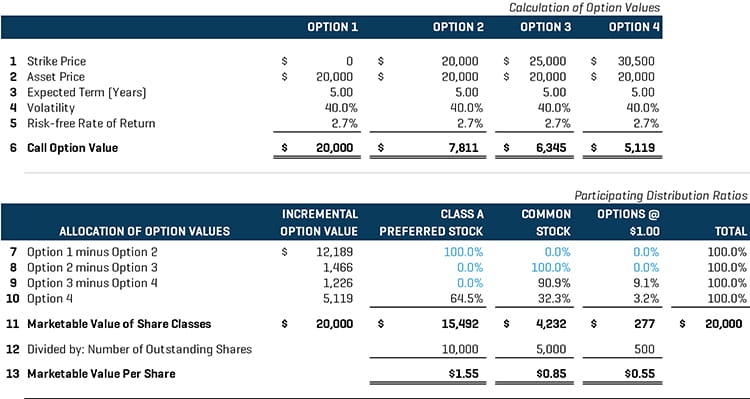On line 1 in Figure 6, we use the strike prices from the breakpoint analysis as the strike prices in a series of Black-Scholes call option analyses. Then, we need to fill the remaining inputs, which are as follows:

• Asset price: Either the equity value or enterprise value of the company;
• Expected term: Term until a potential liquidity event, either IPO or sale of the company, based on management’s input
• Volatility: Equity or asset volatility of the company, which is based on the asset price of the company
• Risk-free rate of return: The risk-free rate of return that corresponds to the assumed term

Based on these inputs, we calculate the call option value for each option and then allocate the incremental option value per added option to the various securities, as presented on lines 7-10 in Figure 6. The allocation of value reflects the seniority characteristics, rights, and privileges of the securities. In detail, the first incremental option value is allocated to the most senior security––Class A preferred stock; the second incremental option value is then allocated to the common stock; next, the options start participating and dilute the common stock holders; finally, all securities participate on an as-converted basis.

### Current Value Method

The CVM assumes an immediate sale of the company, estimates equity value on a controlling basis, and then allocates equity value to various series of preferred stock based on their liquidation preferences. Below, we present a case study on this method.

#### Figure 7. Valuation Analysis – Current Value Method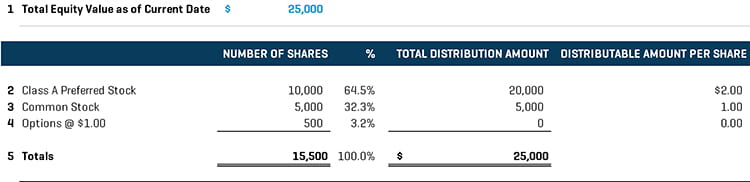The first step is determining the equity value of the company, which is assumed to be \$25.0 million in this case. This equity value is based on either the company’s assessment or an input based on independent analysis that reflects an indication of value assuming a current liquidation of the company. Then, based on the rights and privileges of the securities outstanding, the value is distributed to the Class A preferred stock holders first, then to the common stock holders. If there is a higher indication of value, any incremental value will be allocated on a pro rata basis among the three tranches. If this is the case, then it will be beneficial for the Class A preferred stock to convert into the common stock and the option holders to exercise the options to participate in the upside.

### The Hybrid Method

The hybrid method is a combination of PWERM and OPM and considers multiple future exit scenarios with probabilities. Below, we present a cash study using the hybrid method. We assume an expected one-year term until a liquidity event of either IPO or sale of the company, with a probability of 70% of an IPO event and 30% of a sale event. We also assume the pre-IPO equity value to be \$25 million and the current equity value to be \$20 million. Following the same procedures of PWERM and OPM allocation methods, we obtain the analysis below.

#### Figure 8. PWERM Analysis – IPO Scenario#### Figure 9. Allocation of Equity Value – Sale Scenario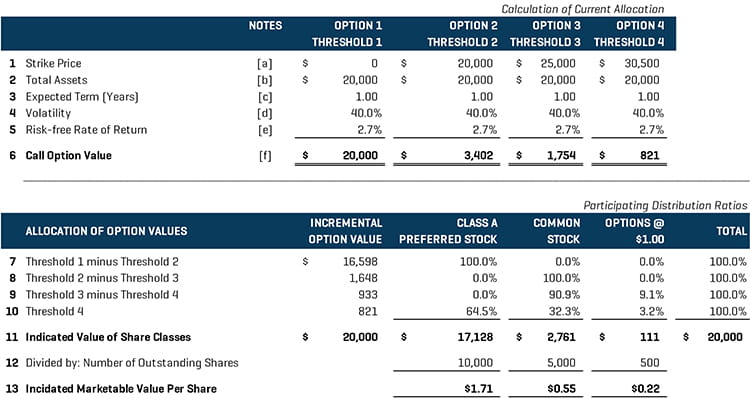Once the equity value is allocated to security classes under both the IPO and sale scenarios, we combine the results in the conclusion and apply the probabilities of occurrence for each scenario.

#### Figure 10. Conclusion of Value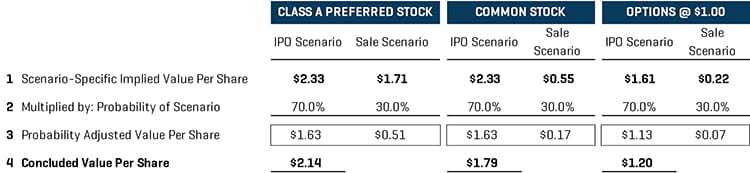From the above example, we realize the appropriateness and usefulness of the hybrid method when we need to consider multiple management exit strategies over a specified period, as well as different valuation assumptions.

### Valuation Method Selection

The Guide provides a helpful flowchart for determining the most appropriate valuation method to use under different circumstances. The flowchart below provides general guidance and an overview of the various factors to consider when selecting a valuation method for equity interests. However, it does not give any consideration to the CVM, which is appropriate to use when there is an immediate liquidity event or the subject interest class has both seniority and control over the exit timing, as described in the previous section. Please refer to the Figure 11 for more details.

#### Figure 11. Valuation Method Selection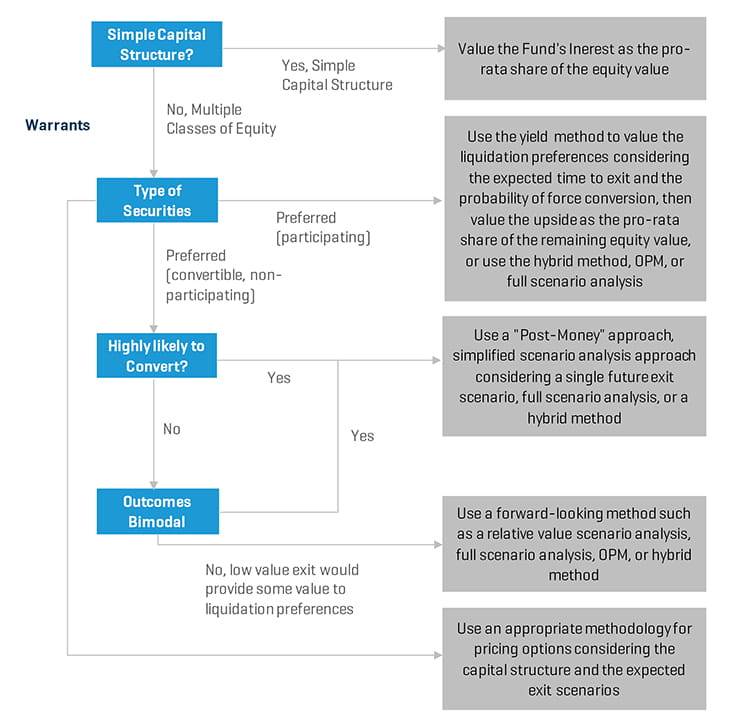The first step is assessing the complexity of the capital structure. Under a simple capital structure, it is appropriate to value the subject interest based on a pro rata share of the underlying equity value of the company. Under a more complex capital structure with multiple classes of equity, the selection of the method depends on the types of the security classes.
When participating preferred stock is included in the capital structure, it is appropriate to value the liquidation preferences of the preferred stock outside of an OPM framework using the yield method with the expected term assumption and value the upside based on a pro rata share or OPM framework using the remaining equity value as an asset price. A hybrid method may be applicable in this case, as well.

When convertible preferred stock is included in the capital structure, the first step is assessing the likelihood of conversion of the preferred stock. If there is a high likelihood of conversion of the preferred stock into common stock, then it is appropriate to apply a post-money approach relying on a simplified scenario analysis, with a single future exit scenario. A full scenario analysis approach or a hybrid method may also be applicable. If the likelihood of conversion into common stock is uncertain, then it is appropriate to use a forward-looking approach such as the OPM framework, a full scenario analysis, or a hybrid method. If newly issued warrants are included in the capital structure, special consideration should be given to the exercise provisions and vesting terms of the warrants when determining the appropriate valuation framework and exit-timing assumptions.

### Critical Points for Valuing Equity Interest

Selecting an appropriate methodology for valuating equity interest in complex capital structures depends largely on the complexity of the actual capital structure, the subject company’s strategic exit plan, and the transaction-specific conditions. As each of the common valuation methods has advantages and disadvantages, it is critical to identify the limitations of the selected method and analyze its suitability for the particular valuation purpose such that the valuation analysis can derive the most appropriate and accurate values. Selecting an inappropriate valuation method may have a tremendous effect on the valuation outcome for any given purpose and may materially underestimate/overestimate either the equity value of the underlying company or subject interest. A careful and elaborate consideration of all the facts and parameters known in relation to the subject company and equity classes – as well as market data, trading observations on secondary markets, open interest for any specific equity classes, and an understanding of the management strategic growth plan and triggering exit milestones – are critical for leading to an objectively and thoroughly supportable valuation method that will fit the valuation requirements in each given case.